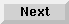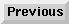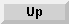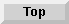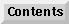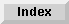10 Matrix Operations

## Matrix Operations

The statements described next use either matrix arrays or vectors.

### MAT . . . CSUM

The sums of all the columns of a matrix can be found by using the MATCSUM statement. Syntax for this statement is as follows:

MAT result vector = CSUM operand matrix

Each element in the result vector is the sum of the corresponding column of the operand matrix.

For example:

```           | 2 5 7 |
Matrix A =
| 9 8 1 |

MAT B = CSUM A

Vector B = | 11  13  8 |
```
The result vector is redimensioned.

### MATRSUM

The sums of all the rows of a matrix can be found by using the MATRSUM statement. Syntax for this statement is as follows:

MAT result vector = RSUM operand matrix

Each element in the result vector is the sum of the corresponding row of the operand matrix.

For example:

```           | 2 4 6 8 |
Matrix A =
| 1 3 5 7 |

MAT C = RSUM A

| 20 |
Vector C =
| 16 |
```
The result vector is redimensioned.

Eloquence Language Manual - 19 DEC 2002